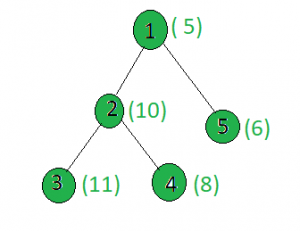# Count the nodes in the given tree whose weight is prime

• Last Updated : 20 Apr, 2021

Given a tree, and the weights of all the nodes, the task is to count the number of nodes whose weight is prime.
Examples:

Input:Output:
Only the weights of the nodes 1 and 3 are prime.

Approach: Perform dfs on the tree and for every node, check if it’s weight is prime or not.
Below is the implementation of above approach:

## C++

 `// C++ implementation of the approach``#include ``using` `namespace` `std;` `int` `ans = 0;` `vector<``int``> graph;``vector<``int``> weight(100);` `// Function that returns true``// if n is prime``bool` `isprime(``int` `n)``{``    ``for` `(``int` `i = 2; i * i <= n; i++)``        ``if` `(n % i == 0)``            ``return` `false``;``    ``return` `true``;``}` `// Function to perform dfs``void` `dfs(``int` `node, ``int` `parent)``{``    ``// If weight of node is prime or not``    ``if` `(isprime(weight[node]))``        ``ans += 1;` `    ``for` `(``int` `to : graph[node]) {``        ``if` `(to == parent)``            ``continue``;``        ``dfs(to, node);``    ``}``}` `// Driver code``int` `main()``{``    ``// Weights of the node``    ``weight = 5;``    ``weight = 10;``    ``weight = 11;``    ``weight = 8;``    ``weight = 6;` `    ``// Edges of the tree``    ``graph.push_back(2);``    ``graph.push_back(3);``    ``graph.push_back(4);``    ``graph.push_back(5);` `    ``dfs(1, 1);` `    ``cout << ans;` `    ``return` `0;``}`

## Java

 `// Java implementation of the approach``import` `java.util.*;` `class` `GFG{`` ` `static` `int` `ans = ``0``;` `static` `Vector[] graph = ``new` `Vector[``100``];``static` `int``[] weight = ``new` `int``[``100``];`` ` `// Function that returns true``// if n is prime``static` `boolean` `isprime(``int` `n)``{``    ``for` `(``int` `i = ``2``; i * i <= n; i++)``        ``if` `(n % i == ``0``)``            ``return` `false``;``    ``return` `true``;``}`` ` `// Function to perform dfs``static` `void` `dfs(``int` `node, ``int` `parent)``{``    ``// If weight of node is prime or not``    ``if` `(isprime(weight[node]))``        ``ans += ``1``;`` ` `    ``for` `(``int` `to : graph[node]) {``        ``if` `(to == parent)``            ``continue``;``        ``dfs(to, node);``    ``}``}`` ` `// Driver code``public` `static` `void` `main(String[] args)``{``    ``for` `(``int` `i = ``0``; i < ``100``; i++)``        ``graph[i] = ``new` `Vector<>();``    ` `    ``// Weights of the node``    ``weight[``1``] = ``5``;``    ``weight[``2``] = ``10``;``    ``weight[``3``] = ``11``;``    ``weight[``4``] = ``8``;``    ``weight[``5``] = ``6``;`` ` `    ``// Edges of the tree``    ``graph[``1``].add(``2``);``    ``graph[``2``].add(``3``);``    ``graph[``2``].add(``4``);``    ``graph[``1``].add(``5``);`` ` `    ``dfs(``1``, ``1``);`` ` `    ``System.out.print(ans);``}``}` `// This code is contributed by Rajput-Ji`

## Python3

 `# Python3 implementation of the approach``ans ``=` `0` `graph ``=` `[[] ``for` `i ``in` `range``(``100``)]``weight ``=` `[``0``] ``*` `100` `# Function that returns true``# if n is prime``def` `isprime(n):``    ``i ``=` `2``    ``while``(i ``*` `i <``=` `n):``        ``if` `(n ``%` `i ``=``=` `0``):``            ``return` `False``        ``i ``+``=` `1``    ``return` `True` `# Function to perform dfs``def` `dfs(node, parent):``    ``global` `ans``    ` `    ``# If weight of the current node is even``    ``if` `(isprime(weight[node])):``        ``ans ``+``=` `1``;``    ` `    ``for` `to ``in` `graph[node]:``        ``if` `(to ``=``=` `parent):``            ``continue``        ``dfs(to, node)` `# Driver code` `# Weights of the node``weight[``1``] ``=` `5``weight[``2``] ``=` `10``weight[``3``] ``=` `11``weight[``4``] ``=` `8``weight[``5``] ``=` `6` `# Edges of the tree``graph[``1``].append(``2``)``graph[``2``].append(``3``)``graph[``2``].append(``4``)``graph[``1``].append(``5``)` `dfs(``1``, ``1``)``print``(ans)` `# This code is contributed by SHUBHAMSINGH10`

## C#

 `// C# implementation of the approach``using` `System;``using` `System.Collections;``using` `System.Collections.Generic;``using` `System.Text;` `class` `GFG{``    ` `static` `int` `ans = 0;``static` `ArrayList[] graph = ``new` `ArrayList;``static` `int``[] weight = ``new` `int``;` `// Function that returns true``// if n is prime``static` `bool` `isprime(``int` `n)``{``    ``for``(``int` `i = 2; i * i <= n; i++)``        ``if` `(n % i == 0)``            ``return` `false``;``            ` `    ``return` `true``;``}` `// Function to perform dfs``static` `void` `dfs(``int` `node, ``int` `parent)``{``    ` `    ``// If weight of node is prime or not``    ``if` `(isprime(weight[node]))``        ``ans += 1;` `    ``foreach``(``int` `to ``in` `graph[node])``    ``{``        ``if` `(to == parent)``            ``continue``;``            ` `        ``dfs(to, node);``    ``}``}``    ` `// Driver Code``public` `static` `void` `Main(``string``[] args)``{``    ``for``(``int` `i = 0; i < 100; i++)``        ``graph[i] = ``new` `ArrayList();``    ` `    ``// Weights of the node``    ``weight = 5;``    ``weight = 10;``    ``weight = 11;``    ``weight = 8;``    ``weight = 6;` `    ``// Edges of the tree``    ``graph.Add(2);``    ``graph.Add(3);``    ``graph.Add(4);``    ``graph.Add(5);` `    ``dfs(1, 1);` `    ``Console.Write(ans);``}``}` `// This code is contributed by rutvik_56`

## Javascript

 ``
Output:
`2`

Complexity Analysis:

• Time Complexity: O(N*sqrt(V)), where V is the maximum weight of a node in the given tree.
In DFS, every node of the tree is processed once and hence the complexity due to the DFS is O(N) when there are N total nodes in the tree. Also, while processing every node, in order to check if the node value is prime or not, a loop up to sqrt(V) is being run, where V is the weight of the node. Hence for every node, there is an added complexity of O(sqrt(V)). Therefore, the time complexity is O(N*sqrt(V)).
• Auxiliary Space: O(1).
Any extra space is not required, so the space complexity is constant.

My Personal Notes arrow_drop_up